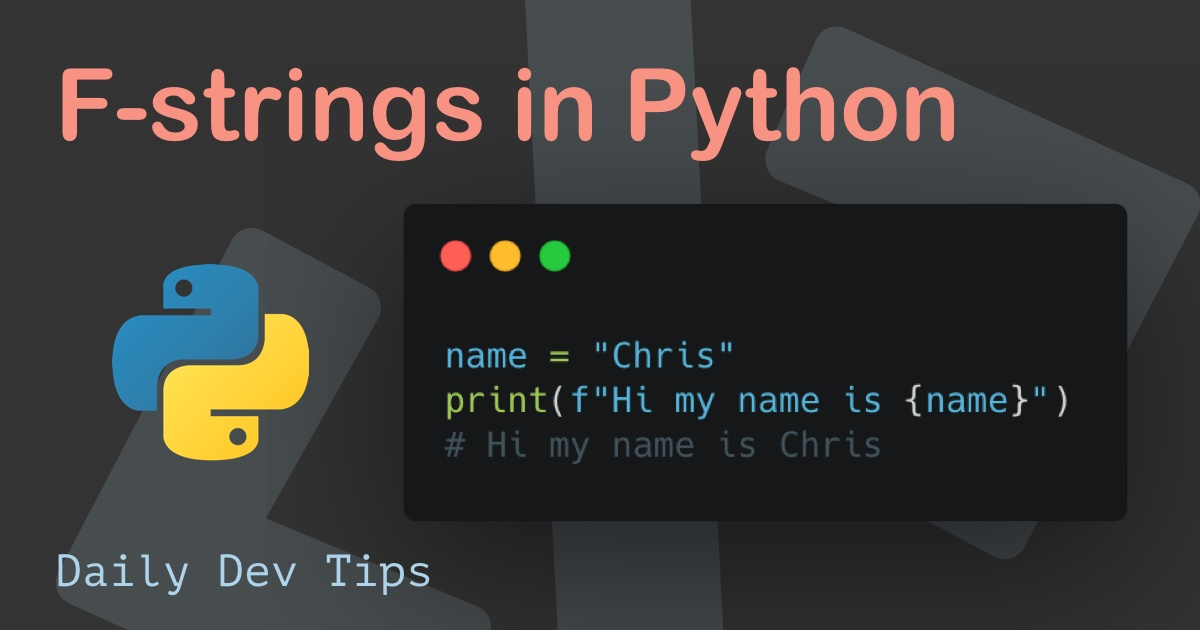Subscribe

# Regular Expressions in Python

••• 2 devs liked it

✍️

Exploring the possibilities for Regular Expressions in Python

30 May, 2021 · 3 min read

Regular Expressions are a touchpoint you find in most programming languages, and there will come a time in your developer life you will need them.

Today I'm going to check out how they work in Python.

Before we can use regular expressions in Python, we have to import the module. It does come with the standard package, so no pip install is needed.

``import re``

There are multiple functions we can use for the Regular Expressions, and they are:

• `findall`: Return a list of all matches
• `search`: Return a match object if there is a match
• `split`: Return a list of split matches
• `sub`: Replace one or more matches with a string

Then there is a whole set of characters and sequences available, which I won't be diving deeper into. I would strongly suggest looking at a regex tool like Pythex.

Let's start by looking at the `findall` function and how it works.

``````import re

string = "he was close to hearing the rain"
x = re.findall("he", string)
print(x)``````

This example will look for all occurrences of `he` and will return a list that states:

``````['he', 'he', 'he']
# he, hearing, the``````

It will return an empty list if nothing is found.

## Python Regular Expression Search function permalink

Another great addition is the search for regular expressions. Let's first see how it works based on the above example.

``````string = "he was close to hearing the rain"
x = re.search("he", string)
print(x)``````

The match object looks something like this:

``<re.Match object; span=(0, 2), match='he'>``

We can use the start and end functions on this result to get that position like this:

``````string = "he was close to hearing the rain"
x = re.search("\s", string)
print("he word starting on position:", x.start(), "and ending on:", x.end())``````

Which will result in:

``he word starting on position: 2 and ending on 3``

## Splitting regular expression results in Python permalink

A quite common approach for RegEx is to split the results into a list. Let's say we want to break all the spaces in a sentence:

``````string = "he was close to hearing the rain"
x = re.split("\s", string)
print(x)``````

Gives us the following list:

``['he', 'was', 'close', 'to', 'hearing', 'the', 'rain']``

We can also give the number of splits we want to make, so we only need the first occurrence.

``````string = "he was close to hearing the rain"
x = re.split("\s", string, 1)
print(x)``````

Gives us:

``['he', 'was close to hearing the rain']``

## Replacing regular expression matches in Python permalink

What we, however, want to do in some cases is replacing specific matches.

Let's say we want to convert all spaces to dashes.

``````string = "he was close to hearing the rain"
x = re.sub("\s", '-', string)
print(x)``````

Will give us the following result:

``he-was-close-to-hearing-the-rain``

Which is super useful for filenames for instance!

Tweet this tip
••• 2 devs liked it

### Speedtest your connection in Python

21 Jun, 2021 · 2 min read### F-strings in Python

7 Jun, 2021 · 2 min readJoin 2103 devs and subscribe to my newsletter

• 1000 articles written
• 2103 devs subscribed
• 529116 words written